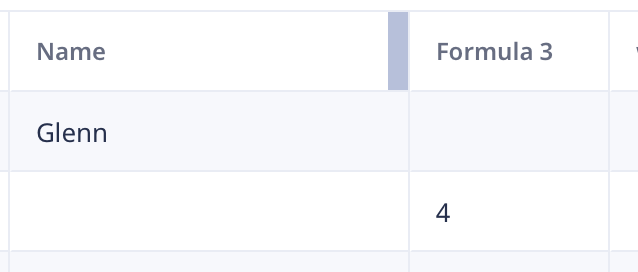0# Dynamic Choice as table list/View column

let's say I have a column 'Quantity' in a table/View. I then add/Show a formula column calculated as:-

``if Quantity = 1 then "one" else "other" end``

In this case, all is correct with 'one' or 'other' displayed in that column, according to the value of Quantity.

However, if I use DC values rather than just text, like:-

``````if Quantity = 1 then DC1 else DC2 end
``````

the column ALWAYS shows DC1. In fact, it doesn't matter which DC field is where, it always shows the first one.

I should point out that both DCs are essentially the same. So same list of options and same selection. They only differ in the display value. If I simply display either/both of them as their own column, they are correct, but not when part of the if… then logic.

I tried using text(DC) and that works, but if there's no choice it shows 'undefined' instead of being blank, so it's a PIA and requires more script to avoid that.

Anyone come across this? A bug perhaps?

3replies Oldest first
• Oldest first
• Popular•• Fred
• Fred
• 5 mths ago
• Reported - view

I tried it on my Mac app and was not able to duplicate. I have this code:

``````if Name = "Glenn" then dChoice else DC1 end
``````

dChoice and DC1 are dynamic choice fields that just happen to point to the same table.

Here is what is looks like in my table:dChoice field is blank for the record with Glenn so Formula 3 is blank. The record with a empty Name field has the 4th record selected in DC1 so that is what shows up in the table.

Like
•• UKenGB
• UKenGB
• 5 mths ago
• Reported - view

Fred Hmm. I'm using the Mac app also. Seems like you're doing the same thing as me, but getting a different result. I'll have to look at this again.

One thing that occurs to me. My DC fields are based on the same table AND both have the same selection/choice. This is different I think from your example above.

The reason for this is that the DC fields point to lists of units (inch, foot, millimetre, metre etc) and the underlying table contains both a 'singular' field and a 'plural' one as I would like the plural to be displayed when Quantity is not 1. Not a big deal, but I like it to be right. So I keep both DC choices the same, but only display the appropriate DC field. On the form, this works fine and as soon as the Quantity changes, the correct DC field will be shown and a different selection can be made if required.

However, in the list/table view I see the problem outlined above. So if the DC1 selection is 'inch' then DC2 would show 'inches' - except only one of them would actually be displayed on the form, depending on the value of Quantity.

Is the problem perhaps because Ninox sees the same table and the same selection, so doesn't bother to get the actual displayed field for the second DC in my if…

``````if Quantity = 1 then DC1 else DC2 end
``````

So in the above scenario, the list/table column would only show 'inch', no matter what the value of Quantity. If I switch DCs, so

``````if Quantity = 1 then DC2 else DC1 end
``````

then every row shows 'inches'. Is Ninox only bothering to get the displayed field of the first DC it sees? Apparently not bothering with the second one on the basis that the record options are the same and so is the selected record. At least, that is what it looks like is occurring.

Like
•• Fred
• Fred
• 5 mths ago
• Reported - view

For background, both dynamic choice fields are pointing to the same table (Table1). So I went back and selected the same choice for both but had a different text display in each and I encountered your issue.

The else part of the if statement does not pull the text displayed from second dC field.

If I put in text before each dynamic choice then it shows the text displayed in the field.

``````if Name = "Glenn" then text(dChoice) else text(DC1) end
``````

You are correct that going this route will give you an "undefined" if no selection is made.

So you could do something like the following to take care of that.

``````if Name = "Glenn" then
if dChoice != null then text(dChoice) else null end
else
if DC1 != null then text(DC1) else null end
end
``````
Like
Like Follow
• 5 mths agoLast active
• 3Replies
• 46Views
• 2 Following